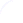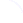Search
 Submission Procedure share: |available in:   PDF (218 kB) PS (249 kB)

get:
 Similar Docs BibTeX Write a comment
get:

DOI:   10.3217/jucs-014-06-0938

### The Bit-Complexity of Finding Nearly Optimal Quadrature Rules for Weighted Integration

Volker Bosserhoff (Universität der Bundeswehr, Germany)

Abstract: Given a probability measure ν and a positive integer n. How to choose n knots and n weights such that the corresponding quadrature rule has the minimum worst-case error when applied to approximate the ν-integral of Lipschitz functions? This question has been considered by several authors. We study this question whithin the framework of Turing machine-based real computability and complexity theory as put forward by [Ko 1991] and others. After having defined the notion of a polynomialtime computable probability measure on the unit interval, we will show that there are measures of this type for which there is no computable optimal rule with two knots. We furthermore characterize - in terms of difficult open questions in discrete complexity theory - the complexity of computing rules whose worst-case error is arbitrarily close to optimal.

Keywords: quadrature rules, real computational complexity

Categories: F.2, F.4.1, G.1.4